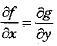# Differential Equationwill have solution of the form

1.  (C1+C2t)e-5t

2.  C1e-2t

3.  C1e-5t+C2e5t

4.  C3e-5t+C2e2t

4

(C1+C2t)e-5t

Explanation :
No Explanation available for this question

# Differential Equation for the variation of the amount of salt x in atank with time t is given by dx/dt+x/20=10 where x is in kg and t is in minutes. Assuming that there is no salt in the tank initially the time (in min)at which the amount of salt in creases to 100kg is

1.  10 In 2

2.  20 In 2

3.  50 In 2

4.  100 In 2

4

20 In 2

Explanation :
No Explanation available for this question

# Solve the differential equation xdx+ydy+2(x2+y2)dx=0

1.  X2-y2=ce-4x

2.  X2+y2=ce4x

3.  X2+y2=ce-4x

4.  X2-y2=ce4x

4

X2+y2=ce-4x

Explanation :
No Explanation available for this question

# The Differential Equationis

1.  First order and Linear

2.  First order and Non-Linear

3.  Second order and Linear

4.  Second order and Non-Linear

4

Second order and Non-Linear

Explanation :
No Explanation available for this question

# For the Differential equation dy/dt+5y=0 with y(0)=1,the general solution is

1.  e5t

2.  e-5t

3.  5e-5t

4.

4

e-5t

Explanation :
No Explanation available for this question

# The Differential Equation y’’+(s3sin x)5y’+y=cos x3 is

1.  Homogeneous

2.  Nonlinear

3.  Second order Linear

4.  Non-homogeneous with constant coefficient

4

Second order Linear

Explanation :
No Explanation available for this question

# For the Differential Equation f(x,y)dy/dx+g(x,y)=0 to be exact

1.

2.

3.  f=g

4.

4Explanation :
No Explanation available for this question

# The Number of Boundary Conditions Required to solve the Following Differential Equationis are

1.  2

2.  4

3.  4

4.  1

4

2

Explanation :
No Explanation available for this question

# Order of the Differential equation is

1.  1

2.  2

3.  3

4.  6

4

2

Explanation :
No Explanation available for this question

1.  1

2.  2

3.  3

4.  6

4